Next: Estimation of Access Costs Up: Query Processing Previous: Other Operations

Estimation of Query-Processing Cost

1. To choose a strategy based on reliable information, the database system may store statistics for each relation r:
•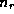- the number of tuples in r.
•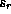- the size in bytes of a tuple of r (for fixed-length records).
• V(A, r) - the number of distinct values that appear in relation r for attribute A.
2. The first two quantities allow us to estimate accurately the size of a Cartesian product.
• The Cartesian product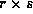contains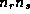tuples.
• Each tuple ofoccupies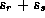bytes.
• The third statistic is used to estimate how many tuples satisfy a selection predicate of the form

<attribute-name> = <value>

• We need to know how often each value appears in a column.
• If we assume each value appears with equal probability, then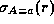is estimated to have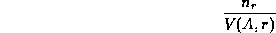tuples.

• This may not be the case, but it is a good approximation of reality in many relations.
• We assume such a uniform distribution for the rest of this chapter.
• Estimation of the size of a natural join is more difficult.
• Let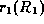and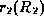be relations on schemes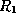and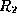.
• If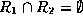(no common attributes), then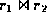is the same as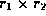and we can estimate the size of this accurately.
• If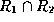is a key for, then we know that a tuple of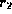will join with exactly one tuple of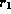.
• Thus the number of tuples inwill be no greater than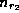.
• Ifis not a key foror, things are more difficult.
• We use the third statistic and the assumption of uniform distribution.
• Assume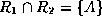.
• We assume there are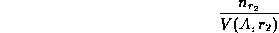tuples inwith an A value of t[A] for tuple t in.

• So tuple t ofproducestuples in3. Considering all the tuples in, we estimate that there are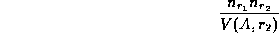tuples in total in4. If we reverse the roles ofandin this equation, we get a different estimate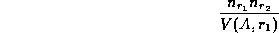if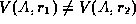.

• If this occurs, there are likely to be some dangling tuples that do not participate in the join.
• Thus the lower estimate is probably the better one.
• This estimate may still be high if the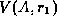values inhave few values in common with the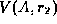values in.
• However, it is unlikely that the estimate is far off, as dangling tuples are likely to be a small fraction of the tuples in a real world relation.
5. To maintain accurate statistics, it is necessary to update the statistics whenever a relation is modified.

This can be substantial, so most systems do this updating during periods of light load on the system.Next: Estimation of Access Costs Up: Query Processing Previous: Other Operations

Osmar Zaiane
Sun Jul 26 17:45:14 PDT 1998